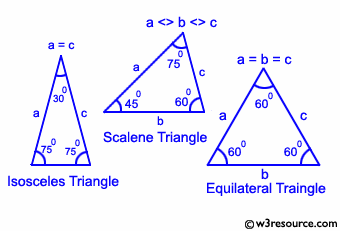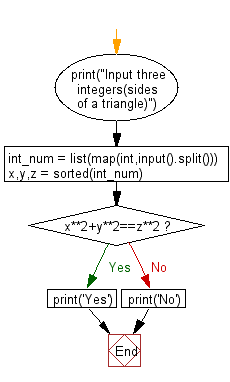﻿ Python: Check whether three given lengths of three sides form a right triangle - w3resource# Python: Check whether three given lengths of three sides form a right triangle

## Python Basic - 1: Exercise-34 with Solution

Write a Python program to check whether three given lengths (integers) of three sides form a right triangle. Print "Yes" if the given sides form a right triangle otherwise print "No".

Input:
Integers separated by a single space.
1 ≤ length of the side ≤ 1,000

Pictorial Presentation:Sample Solution:

Python Code:

``````print("Input three integers(sides of a triangle)")
int_num = list(map(int,input().split()))
x,y,z = sorted(int_num)
if x**2+y**2==z**2:
print('Yes')
else:
print('No')
``````

Sample Output:

```Input three integers(sides of a triangle)
8 6 7
No
```

Flowchart:Python Code Editor:

Have another way to solve this solution? Contribute your code (and comments) through Disqus.

What is the difficulty level of this exercise?

Test your Programming skills with w3resource's quiz.

﻿

## Python: Tips of the Day

Iterating over dictionaries using 'for' loops:

I am a bit puzzled by the following code: d = {'x': 1, 'y': 2, 'z': 3} for key in d: print key, 'corresponds to', d[key] What I don't understand is the key portion. How does Python recognize ...

key is just a variable name.

```for key in d:
```

For Python 3.x:

```for key, value in d.items():
```

For Python 2.x:

```for key, value in d.iteritems():
```

To test for yourself, change the word key to poop.

In Python 3.x, iteritems() was replaced with simply items(), which returns a set-like view backed by the dict, like iteritems() but even better. This is also available in 2.7 as viewitems().

The operation items() will work for both 2 and 3, but in 2 it will return a list of the dictionary's (key, value) pairs, which will not reflect changes to the dict that happen after the items() call. If you want the 2.x behavior in 3.x, you can call list(d.items()).

Ref: https://bit.ly/37dm0Qo